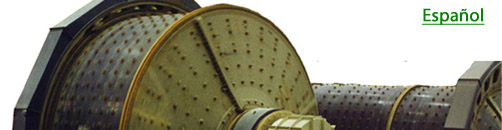expertise in mineral grinding systemsIssue No. 2                                                                                    May, 2012Quickly Determine Your Fineness Of Grind

When flotation (or other mineral separation) is not performing well and you suspect the grind may be the cause, there is a quick way to check it using only a container with a fixed volume (such as a one liter Marcy cup), a weigh scale and a single sieve. The best way to demonstrate this is with an example.

Sylvie, the Plant Metallurgist, first collects grinding circuit cyclone overflow slurry into a one liter Marcy cup. She weighs it, subtracts the weight of the cup, and determines the weight of the slurry to be 1,200 gms. The slurry SG then is 1.2. Knowing the ore has an SG of 3.0, she calculates the weight of solids in the cup as follows.

 Weight (gms) SG Volume (cc) Solids (ore) A? 3.0 B? Water C? 1.0 C? Slurry (total) 1,200 1.2 1,000 A = 1,200 - C C = 1,000 - B Therefore A = 1,200 - (1,000 - B) = 200 +B

Also, A = B x 3. Therefore 200 + B = 3 x B, or 200 = 2 x B. Thus B = 100. Then A = 200 + B = 300. The weight of the solids in the cup thus calculates out to be 300 gms.

Sylvie rinses out the cup over a 65 mesh (212 um) sieve mounted on a vibrating ring, washing until the retained material is clean. She returns the sieve oversize to the Marcy cup and fills it to the top with water, weighs it, subtracts the weight of the cup, this time obtaining 1,080 gms. of slurry. Again she calculates the weight of solids in the cup as follows.

 Weight (gms) SG Volume (cc) Solids (ore) A? 3.0 B? Water C? 1.0 C? Slurry (total) 1,080 1.08 1,000 A = 1,080 - C C = 1,000 - B Therefore A = 1,080 - (1,000 - B) = 80 +B

Also, A = B x 3. Therefore 80 + B = 3 x B, or 80 = 2 x B. Thus B = 40. Then A = 80 + B = 120. The weight of the plus 212 um solids in the cup thus calculates out to be 120 gms.

Therefore, the original sample taken was (120/300 x 100 =) 40% retained (and 60% passing) 212 um.

Since the target grind at Sylvie's plant is 80% passing 212 um, the grind indeed is significantly off and the cause of the downstream separation problems.

While this procedure takes only a few minutes, is not very accurate. In a variation of this procedure, Mr. Barry Wills (please see the reference, below, for which we graciously acknowledge Mr. Wills) has shown a likely variation of plus or minus 5% passing the selected sieve opening size compared to a full laboratory screen analysis procedure. This can be partially addressed by practicing and fine tuning the steps, and by repeat measurements.

Reference: Wills, B.A., 1988, "A Rapid Method for Measurement of Fineness of Grind", Minerals Engineering, Vol.1, No. 1, pp. 81-84.